# Convective Heat Transfer: One dimensional (Part - 3) Chemical Engineering Notes | EduRev

## Chemical Engineering : Convective Heat Transfer: One dimensional (Part - 3) Chemical Engineering Notes | EduRev

The document Convective Heat Transfer: One dimensional (Part - 3) Chemical Engineering Notes | EduRev is a part of the Chemical Engineering Course Heat Transfer for Engg..
All you need of Chemical Engineering at this link: Chemical Engineering

3.2.2 Heat transfer between fluids separated by a cylindrical wall
In the above section we have seen that how the rate of heat transfer is calculated when the two fluids are separated by a flat wall. Another commonly encountered shape in the chemical engineering plant is the heat transfer between fluids separated by a cylindrical wall. Therefore, we will see them to understand the overall heat transfer coefficient in such a system. Consider a double pipe heat exchanger which consists of two concentric pipes arrange as per the fig. 3.4.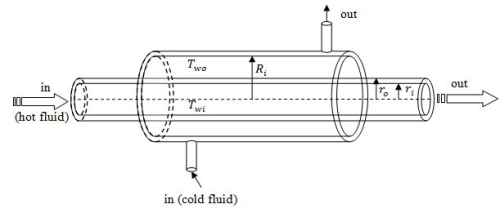Fig. 3.5: Schematic of a co-current double pipe heat exchanger

The purpose of a heat exchanger is to increase the temperature of a cold fluid and decrease that of the hot fluid which is in thermal contact, in order to achieve heat transfer.

The fig. 3.5 shows that the hot fluid passes through the inner tube and the cold fluid passes through the outer tube of the double pipe heat exchanger. The inner and the outer radii of the inner pipe are ri and ro  , respectively, whereas the inner radius of the outer tube is RThe heat transfer coefficient of the fluid in the inner pipe is  hand the heat transfer coefficient of the fluid over the inner pipe is ho. Twi and Two are the inner and outer wall temperatures of the inner pipe. The bulk fluid temperatures of the hot and cold fluids are To and Ti , respectively, at steady state condition and assumed to be fairly constant over the length of the pipe (say L). The construction in fig. 3.6 provides a better understanding.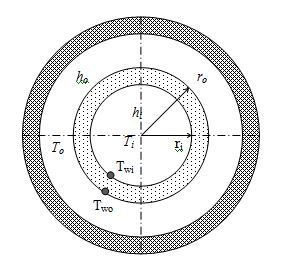Fig. 3.6: Cross-section of the double pipe heat exchanger shown in fig. 3.5

The rate of heat transfer from the hot fluid to the inner surface which is at temperature Twi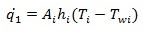(3.12)

The rate of heat transfer through the pipe wall is,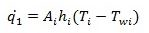(3.13)

(Refer to the section, heat conduction through varying area.)

The rate of heat transfer from the outer surface of the inner pipe to the cold fluid is,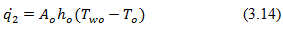The rate of heat transfers will be same, thus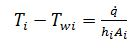(3.15)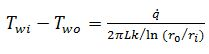(3.16)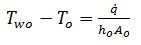(3.17)

Thus on rearranging above equations,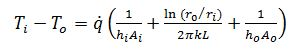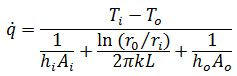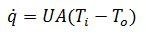(3.18)

where,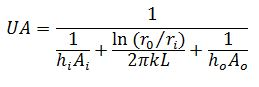If we compare the overall heat transfer coefficient shown above with the overall heat transfer coefficient discussed in eq.3.11 (for flat plate). It can be seen that due to the different inside and outside radii of the pipe, the overall heat transfer coefficient will be different. Therefore, the overall heat transfer coefficient can be defined either by Ui (overall heat transfer coefficient based on inside surface area) or Uo (overall heat transfer coefficient based on outside surface area). But it should be noted that the rate of heat transfer and the driving force remain the same. Therefore, we have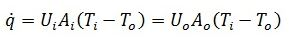(3.19)

where,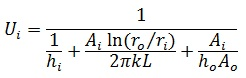or,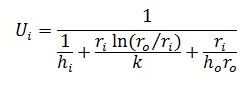Similarly,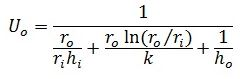In terms of thermal resistance, we can use eq. 3.19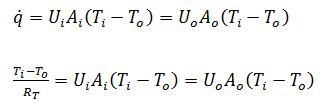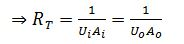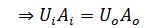(3.20)

Illustration 3.2.

Warm methanol flowing in the inner pipe of a double pipe heat exchanger is being cooled by the flowing water in the outer tube of the heat exchanger. The thermal conductivity of the exchanger, inner and outer diameter of the inner pipe are 45 W/(m·oC), 26 mm, and 33 mm, respectively. The individual heat transfer coefficients are:

Coefficient (W/(m2·oC))

Methanol, hi         1000

Water, ho               1750

Calculate the overall heat transfer coefficient based on the outside area of the inner tube.

Solution 3.2

Using following equation,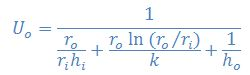It is apparent that all the values are known. Thus, on putting the values theUis 519 W/(m2·oC).

Offer running on EduRev: Apply code STAYHOME200 to get INR 200 off on our premium plan EduRev Infinity!

## Heat Transfer for Engg.

46 videos|60 docs|59 tests

,

,

,

,

,

,

,

,

,

,

,

,

,

,

,

,

,

,

,

,

,

;